## Addition of Angular Momentum

It is often required to add angular momentum from two (or more) sources together to get states of definite total angular momentum. For example, in the absence of external fields, the energy eigenstates of Hydrogen (including all the fine structure effects) are also eigenstates of total angular momentum. This almost has to be true if there is spherical symmetry to the problem.

As an example, lets assume we are adding the orbital angular momentum from two electrons,and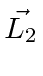to get a total angular momentum. We will show that the total angular momentum quantum number takes on every value in the range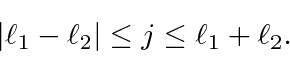We can understand this qualitatively in the vector model pictured below. We are adding two quantum vectors.The length of the resulting vector is somewhere between the difference of their magnitudes and the sum of their magnitudes, since we don't know which direction the vectors are pointing.

The states of definite total angular momentum with quantum numbers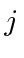and, can be written in terms of products of the individual states (like electron 1 is in this state AND electron 2 is in that state). The general expansion is called the Clebsch-Gordan series:or in terms of the ket vectorsThe Clebsch-Gordan coefficients are tabulated although we will compute many of them ourselves.

When combining states of identical particles, the highest total angular momentum state,, will always be symmetric under interchange.The symmetry under interchange will alternate asis reduced.

The total number of states is always preserved. For example if I add two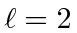states together, I get total angular momentum states with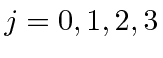and 4. There are 25 product states since eachstate has 5 different possibles. Check that against the sum of the number of states we have just listed.where the numbers are the number of states in the multiplet.

We will use addition of angular momentum to:

• Add the orbital angular momentum to the spin angular momentum for an electron in an atom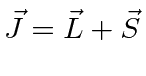;
• Add the orbital angular momenta together for two electrons in an atom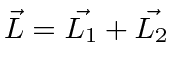;
• Add the spins of two particles together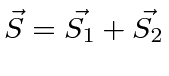;
• Add the nuclear spin to the total atomic angular momentum;
• Add the total angular momenta of two electrons together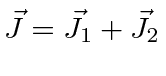;
• Add the total orbital angular momentum to the total spin angular momentum for a collection of electrons in an atom;
• Write the product of spherical harmonics in terms of a sum of spherical harmonics.

Jim Branson 2013-04-22